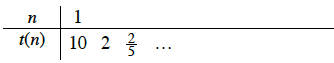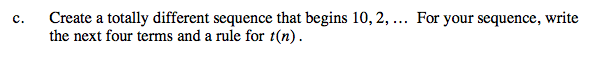### Home > AC > Chapter 13 > Lesson 13.RE3-S > Problem2-156

2-156.
1. Consider the sequence 10, 2, … Homework Help ✎

1. Assuming that the sequence is arithmetic with t(1) as the first term, write the next four terms of the sequence and then write a rule for t(n).

2. Assuming that the sequence is geometric with t(1) as the first term, write the next four terms of the sequence and then write a rule for t(n).

3. Create a totally different sequence that begins 10, 2, … For your sequence, write the next four terms and a rule for t(n).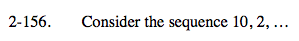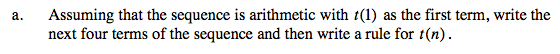Refer to the Math Notes box in Lesson 2.1.8, shown below.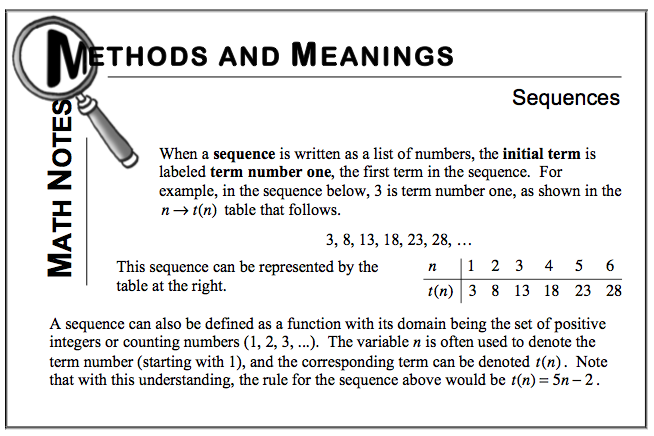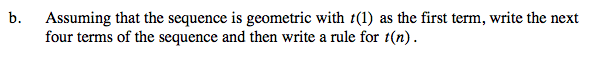Try copying and completing the table below: